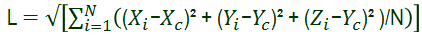# Large Displacement Solution

The linear theory assumes small displacements. It also assumes that the normals to contact areas do not change direction during loading. Hence, it applies the full load in one step. This approach may lead to inaccurate results or convergence difficulties in cases where these assumptions are not valid.

You can request large displacement solution. A large displacement solution takes more time and resources than the small displacement solution but gives more accurate results. The large displacement solution is needed when the acquired deformation alters the stiffness (ability of the structure to resist loads) significantly. The small displacement solution assumes that the stiffness does not change during loading. The large displacement solution assumes that the stiffness changes during loading so it applies the load in steps and updates the stiffness for each solution step.

When the Large displacement option in the Static dialog box is activated, the static study is solved by stepping the load and updating the geometry.

When using large displacement solution for static studies, you can only view the results at the last step which corresponds to the full load. In nonlinear studies, you can view the results of each solution step.
When you run a static analysis and all the displacements are calculated, the solver performs a large displacement check. It issues a warning message when excessive displacements are calculated. The solver performs the following checks:
• For models with Pin connectors, verifies that the relative rotation (θ) within each individual Pin connector is small. The solver issues a warning message when the relative rotation θ is larger than 5o (degrees).
• For all models, checks the maximum displacement (Euclidean norm) among the total number of nodes and compares it to the characteristic length of the model. If the ratio of the maximum displacement over the characteristic length of the model is larger than 10%, the solver issues a warning message. The characteristic length of the model (L) is calculated as:where: Xi, Yi, and Zi are the coordinates of node i; X c, Yc, and Zc are the coordinates of the model geometric center; and N is the total number of nodes in the model.

To activate the Large displacement option:

1. In the Simulation study tree, right-click the static study icon and select Properties.

The Static dialog box appears.

2. On the Options tab, check Large displacement.
3. Click OK.

This option dictates how the software proceeds with the contact problem. For an example of this function, See the Online Tutorial.

 Option Unchecked The full load is applied at once. Source and target pairs are set based on the initial configuration and remain unchanged during contact iterations. Normals to contact areas are also based on the initial configuration and remain unchanged during contact. This approach can lead to inaccurate results or convergence difficulties if these assumptions are not valid, a likely situation when displacements become large. Option Checked Loads are applied gradually and uniformly in a number of steps up to their full values. The number of steps are decided internally by the program based on deformation results. Source and target pairs and normals to contact areas are evaluated at each solution step.

## Recommended Procedure

The following procedure is recommended for solving contact problems:

1. Solve the problem without activating the Large displacement option.
2. Activate the Large displacement option and try again in the following cases:
• If displacements or the orientations of the contacting faces are noticeable when the deformed shape is plotted using a scale factor of 1.0.
• If the highest strain exceeds 4%.
• If von Mises stresses are close to yield.

## Notes

• Full nonlinear analysis is required to handle large strain and material nonlinearity.
• The large displacement solution does not work with:
• Spot weld and bearing connectors
• Beam mesh or mixed mesh that includes beams